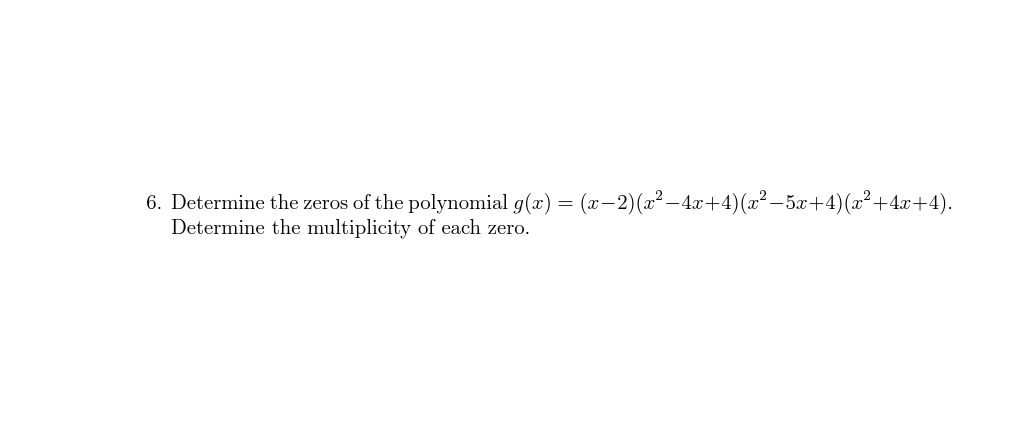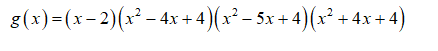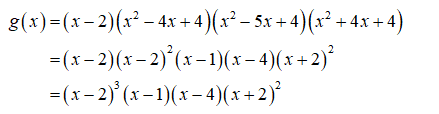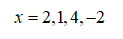# 6. Determine the zeros of the polynomial g(x) = (x-2)(x² – 4.x+4)(x² – 5x+4)(x²+4x+4).Determine the multiplicity of each zero.

Question
1 viewshelp_outlineImage Transcriptionclose6. Determine the zeros of the polynomial g(x) = (x-2)(x² – 4.x+4)(x² – 5x+4)(x²+4x+4). Determine the multiplicity of each zero. fullscreen
check_circle

Step 1

The given polynomial isStep 2

The given function can be rewritten as shown below:Step 3

From the above equation, the zeroes of the given polynomial can be obtained as shown below:...

### Want to see the full answer?

See Solution

#### Want to see this answer and more?

Solutions are written by subject experts who are available 24/7. Questions are typically answered within 1 hour.*

See Solution
*Response times may vary by subject and question.
Tagged in